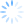Login/Register Math Worksheets, Activities, Games Helpline 099826-99306

# Multiplication math activity worksheet - Multiplication facts

Games are the fastest way to teach and to learn. We got the idea for this multiplication activity worksheet while playing with young students in the class. When kids play, they work at amazing speeds. They understand the situation quickly and are ready to apply the rules immediately in the game play. We leveraged our dice game here to connect math and multiplication with game. This multiplication worksheet addresses multiplication facts and multiplication as repeated addition through activiies

Print out this math activity worksheet on multiplication facts. Kids have to write the multiplication facts and also draw them on the dice. We have used an ancient Indian dice game of chausar as a backdrop and have tried to build from there upwards. Give it a try. They would love to draw out the multiplication facts and it would be the easiest way to recall them in future.The objective of this math ACTIVITY worksheet is to leverage activity and games. Games generate interest and activities help in massively improving recall. When we DO something, our ability to recall and retain is up to 47 times more. Multiplication facts have traditionally been the hardest and most boring of the lot that math expects us to do. Hopefully, this math activity worksheet will provide some help. For more math worksheets on multiplication facts and other topics, use the following links - math worksheets for grade 1, more math worksheets for all classes